For which of the following functions f is f(x) = f(1-x) for all x?

A. f(x) = 1 - x

B. f(x) = 1 -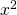C. f(x) =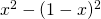D. f(x) =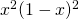E. f(x) = 1/(1-x)

GMAT tutoring students often dislike these function questions. They see f(x) and the brain shuts down: pinwheel of doom (on mac) or BSOD (blue screen of death) on windows. I think it's tough to grasp exactly what you need to do from the first read. That's OK and an important thing to get used to. Go back and read it again. Think. Jot some things down. Challenge yourself. I think you'll find most of these function questions are rather basic. No complicated math. No tricks. Ok, so what does f(x) = f(1-x) mean?

We just need to find the answer choice for which plugging in x yields the same value as plugging in 1 - x. Let's say x is 4. Then the function must yield the same value for 4 as for 1 - 4 (-3). Easy? It really is that easy. For this style of function question pick numbers. I'd probably avoid 1 (just my own superstition) but pretty much anything is fair game. Let's say X is 3. Let's go through the answers and find the one for which plugging in 3 and 1 - 3 gives the same value. You can also find a video explanation here: For which of the following functions f is f(x) = f(1-x) for all x?

Also, to start more basic and to see a bunch of worked through examples here's our main post on GMAT Functions.

A. f(x) = 1 - x

x = 3

1-3 = -2

x = -2

1-(-2) = 3

B. f(x) = 1 -x = 3

1-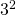= -8

x = -2

1 -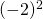= -3

C. f(x) =x = 3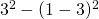= 5

x = -2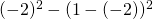= -5

D. f(x) =x = 3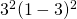= 36

x = -2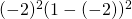= 36

E. f(x) = 1/(1-x)

x = 3

1/(1-3) = -1/2

x = -2

1/(1-(-2)) = 1/3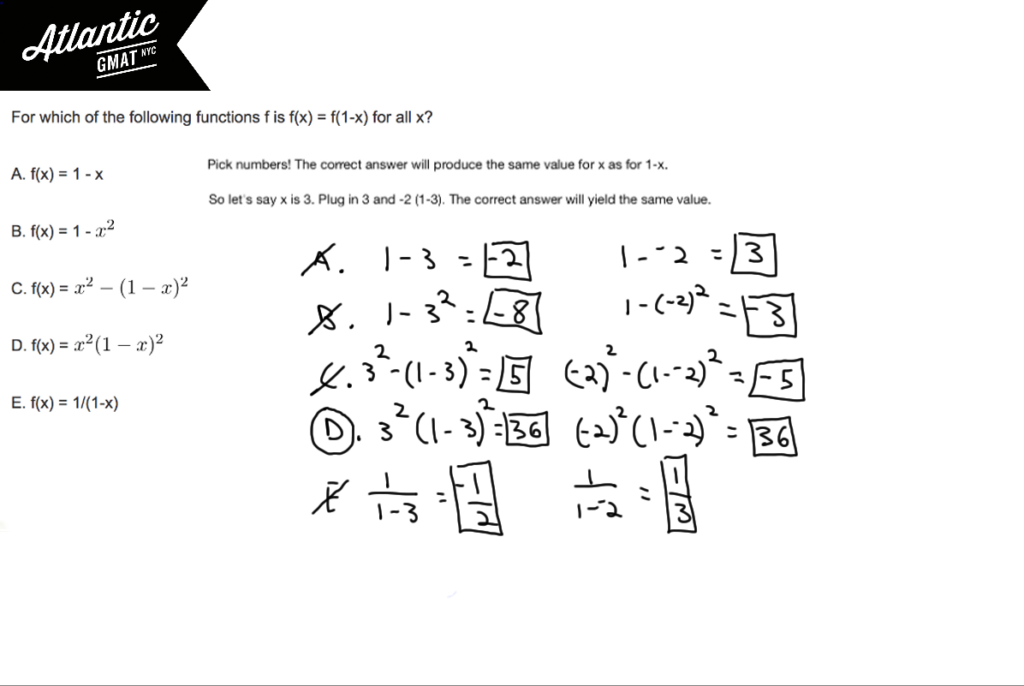This function question is relatively common. Usually plugging in numbers makes it easy to solve. Here are two more GMAT Function questions. The first one is very similar to this one while the second adds some number properties to the mix and is on of the most challenging questions in the GMAT universe:

For which of the following functions is f(a+b) = f(b) + f(a) for all positive numbers a and b?

For every positive even integer n the function h(n) is defined to be the product

Remember: Don't rush the follow through. Do your arithmetic carefully. That doesn't mean quadruple check everything. Just go at the pace that makes sense for you so that you can produce accurate calculations consistently. Comment away with any questions or concerns!

# CONTACT

Atlantic GMAT

405 East 51st St.

NY, NY 10022

(347) 669-3545

info@AtlanticGMAT.com